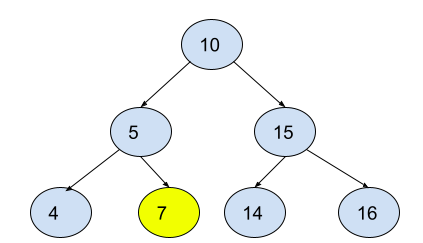# Kth smallest node in BST

Posted: 2 Dec, 2020
Difficulty: Moderate

## PROBLEM STATEMENT

#### You have been given a Binary Search Tree of integers. You are supposed to return the k-th (1-indexed) smallest element in the tree.

##### For example:
``````For the given binary search tree and k = 3
````````````The 3rd smallest node is highlighted in yellow colour.
``````
##### Input Format:
``````The first line contains an integer 'T' which denotes the number of test cases or queries to be run. Then the test cases are as follows.
The first line of each test case contains an integer that denotes the ‘k’ as described in the problem statement.
The second line of each test case contains elements of the tree in the level order form. The line consists of values of nodes separated by a single space. In case a node is null, we take -1 in its place.

For example, the input for the tree depicted in the below image would be :
````````````1
2 3
4 -1 5 6
-1 7 -1 -1 -1 -1
-1 -1
``````

#### Explanation :

``````Level 1 :
The root node of the tree is 1

Level 2 :
Left child of 1 = 2
Right child of 1 = 3

Level 3 :
Left child of 2 = 4
Right child of 2 = null (-1)
Left child of 3 = 5
Right child of 3 = 6

Level 4 :
Left child of 4 = null (-1)
Right child of 4 = 7
Left child of 5 = null (-1)
Right child of 5 = null (-1)
Left child of 6 = null (-1)
Right child of 6 = null (-1)

Level 5 :
Left child of 7 = null (-1)
Right child of 7 = null (-1)

The first not-null node(of the previous level) is treated as the parent of the first two nodes of the current level. The second not-null node (of the previous level) is treated as the parent node for the next two nodes of the current level and so on.
The input ends when all nodes at the last level are null(-1).
``````
##### Note :
``````The above format was just to provide clarity on how the input is formed for a given tree.
The sequence will be put together in a single line separated by a single space. Hence, for the above-depicted tree, the input will be given as:

1 2 3 4 -1 5 6 -1 7 -1 -1 -1 -1 -1 -1
``````
##### Output Format:
``````Print an integer which denotes the K-th smallest node in the given binary search tree.

For each test case, print the output in a separate line.
``````
##### Note :
``````You do not need to print anything; it has already been taken care of.
``````
##### Constraints:
``````1 <= T <= 50
1 <= N <= 10000
1 <= K <= N
-10^8 <= data <= 10^8 and data != -1

Where ‘T’ is the number of test cases, and ‘N’ is the total number of nodes in the binary search tree, ‘K’ is the given integer and “data” is the value of the binary search tree node.

Time Limit: 1sec
``````Approach 1

The basic idea of this approach is to do an in-order traversal of the given BST and store the nodes in an array/list which will be sorted in non-decreasing order. The (k - 1)-th index element of that array/list will be the k-th smallest node.

Let us define a recursive function

void inorder(TreeNode<int> *root, vector<int> &nodes)

which does the inorder traversal of the given BST and stores the nodes of BST in the “nodes”.

Now consider the following steps to implement the function.

1. Base case: If the root node is NULL, then return.
2. Recur for the left-subtree i.e. inorder(root->left, nodes)
3. Store the current node value in the “nodes” array/list
4. Recur for the right-subtree i.e. inorder(root->right, nodes)

We can get the k-th smallest node from the “nodes” array/list. It will be on (k - 1)th index.